## C# || How To Find The Minimum Time To Collect All Apples In A Tree Using C#The following is a module with functions which demonstrates how to find the minimum time to collect all apples in a tree using C#.

1. Min Time – Problem Statement

Given an undirected tree consisting of n vertices numbered from 0 to n-1, which has some apples in their vertices. You spend 1 second to walk over one edge of the tree. Return the minimum time in seconds you have to spend to collect all apples in the tree, starting at vertex 0 and coming back to this vertex.

The edges of the undirected tree are given in the array edges, where edges[i] = [ai, bi] means that exists an edge connecting the vertices ai and bi. Additionally, there is a boolean array hasApple, where hasApple[i] = true means that vertex i has an apple; otherwise, it does not have any apple.

Example 1:``` Input: n = 7, edges = [[0,1],[0,2],[1,4],[1,5],[2,3],[2,6]], hasApple = [false,false,true,false,true,true,false] Output: 8 Explanation: The figure above represents the given tree where red vertices have an apple. One optimal path to collect all apples is shown by the green arrows. ```

Example 2: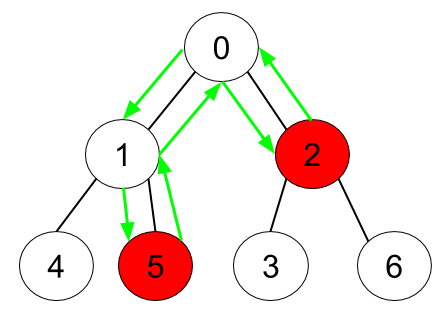``` Input: n = 7, edges = [[0,1],[0,2],[1,4],[1,5],[2,3],[2,6]], hasApple = [false,false,true,false,false,true,false] Output: 6 Explanation: The figure above represents the given tree where red vertices have an apple. One optimal path to collect all apples is shown by the green arrows. ```

Example 3:

``` Input: n = 7, edges = [[0,1],[0,2],[1,4],[1,5],[2,3],[2,6]], hasApple = [false,false,false,false,false,false,false] Output: 0 ```

2. Min Time – Solution

The following is a solution which demonstrates how to find the cheapest flights within K stops.

``` 2. Min Time - Solution C# // ============================================================================ // Author: Kenneth Perkins // Date: May 23, 2023 // Taken From: http://programmingnotes.org/ // File: Solution.cs // Description: Demonstrates how to find minimum time to collect apples // ============================================================================ public class Solution { public int MinTime(int n, int[][] edges, IList<bool> hasApple) { var adj = new Dictionary<int, List<int>>(); foreach (int[] edge in edges) { int a = edge; int b = edge; if (!adj.ContainsKey(a)) { adj[a] = new List<int>(); } if (!adj.ContainsKey(b)) { adj[b] = new List<int>(); } adj[a].Add(b); adj[b].Add(a); } return DFS(0, -1, adj, hasApple); } public int DFS(int node, int parent, Dictionary<int, List<int>> adj, IList<bool> hasApple) { if (!adj.ContainsKey(node)) { return 0; } int totalTime = 0; foreach (int child in adj[node]) { if (child == parent) { continue; } int childTime = DFS(child, node, adj, hasApple); // childTime > 0 indicates subtree of child has apples. Since the root node of the // subtree does not contribute to the time, even if it has an apple, we have to check it // independently. if (childTime > 0 || hasApple[child]) { totalTime += childTime + 2; } } return totalTime; } }// http://programmingnotes.org/ 12345678910111213141516171819202122232425262728293031323334353637383940414243444546 // ============================================================================//    Author: Kenneth Perkins//    Date:   May 23, 2023//    Taken From: http://programmingnotes.org///    File:  Solution.cs//    Description: Demonstrates how to find minimum time to collect apples// ============================================================================public class Solution {    public int MinTime(int n, int[][] edges, IList<bool> hasApple) {        var adj = new Dictionary<int, List<int>>();        foreach (int[] edge in edges) {            int a = edge;            int b = edge;            if (!adj.ContainsKey(a)) {                adj[a] = new List<int>();            }            if (!adj.ContainsKey(b)) {                adj[b] = new List<int>();            }            adj[a].Add(b);            adj[b].Add(a);        }        return DFS(0, -1, adj, hasApple);    }     public int DFS(int node, int parent, Dictionary<int, List<int>> adj, IList<bool> hasApple) {        if (!adj.ContainsKey(node)) {            return 0;        }         int totalTime = 0;        foreach (int child in adj[node]) {            if (child == parent) {                continue;            }            int childTime = DFS(child, node, adj, hasApple);            // childTime > 0 indicates subtree of child has apples. Since the root node of the            // subtree does not contribute to the time, even if it has an apple, we have to check it            // independently.            if (childTime > 0 || hasApple[child]) {                totalTime += childTime + 2;            }        }        return totalTime;    }}// http://programmingnotes.org/ ```

QUICK NOTES:
The highlighted lines are sections of interest to look out for.

The code is heavily commented, so no further insight is necessary. If you have any questions, feel free to leave a comment below.

Once compiled, you should get this as your output for the example cases:

``` 8 6 0 ```

## C# || How To Find The Maximum Profit In Job Scheduling Using C#The following is a module with functions which demonstrates how to find the maximum profit in job scheduling using C#.

1. Job Scheduling – Problem Statement

We have n jobs, where every job is scheduled to be done from startTime[i] to endTime[i], obtaining a profit of profit[i].

You’re given the startTime, endTime and profit arrays, return the maximum profit you can take such that there are no two jobs in the subset with overlapping time range.

If you choose a job that ends at time X you will be able to start another job that starts at time X.

Example 1: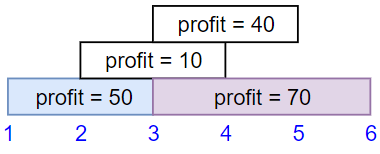``` Input: startTime = [1,2,3,3], endTime = [3,4,5,6], profit = [50,10,40,70] Output: 120 Explanation: The subset chosen is the first and fourth job. Time range [1-3]+[3-6] , we get profit of 120 = 50 + 70. ```

Example 2: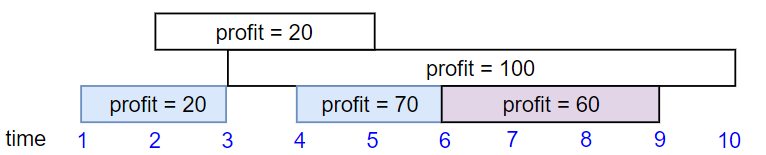``` Input: startTime = [1,2,3,4,6], endTime = [3,5,10,6,9], profit = [20,20,100,70,60] Output: 150 Explanation: The subset chosen is the first, fourth and fifth job. Profit obtained 150 = 20 + 70 + 60. ```

Example 3: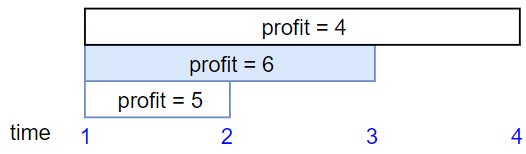``` Input: startTime = [1,1,1], endTime = [2,3,4], profit = [5,6,4] Output: 6 ```

2. Job Scheduling – Solution

The following is a solution which demonstrates how to find the maximum profit in job scheduling.

``` 2. Job Scheduling - Solution C# // ============================================================================ // Author: Kenneth Perkins // Date: Jan 1, 2023 // Taken From: http://programmingnotes.org/ // File: Solution.cs // Description: Demonstrates how to find maximum profit in job scheduling // ============================================================================ public class Solution { public int JobScheduling(int[] startTime, int[] endTime, int[] profit) { if (startTime == null || startTime.Length == 0) { return 0; } var jobs = new List<Job>(); for (int index = 0; index < startTime.Length; ++index) { jobs.Add(new Job(startTime[index], endTime[index], profit[index])); } jobs.Sort((a, b) => a.Start - b.Start); return DFS(jobs, 0, new Dictionary<int, int>()); } private int DFS(List<Job> jobs, int currentJobIndex, Dictionary<int, int> maxProfits) { if (currentJobIndex >= jobs.Count) { return 0; } if (maxProfits.ContainsKey(currentJobIndex)) { return maxProfits[currentJobIndex]; } int next = BinarySearch(jobs, currentJobIndex); int inc = jobs[currentJobIndex].Profit + (next == -1 ? 0 : DFS(jobs, next, maxProfits)); int exc = DFS(jobs, currentJobIndex + 1, maxProfits); int maxProfit = Math.Max(inc, exc); maxProfits[currentJobIndex] = maxProfit; return maxProfit; } private int BinarySearch(List<Job> jobs, int currentJobIndex) { int lo = currentJobIndex; int high = jobs.Count - 1; int result = -1; while (lo <= high) { int mid = lo + (high - lo) / 2; if (jobs[currentJobIndex].End <= jobs[mid].Start) { result = mid; high = mid - 1; } else { lo = mid + 1; } } return result; } class Job { public int Start { get; set; } public int End { get; set; } public int Profit { get; set; } public Job(int startTime, int endTime, int profit) { Start = startTime; End = endTime; Profit = profit; } } }// http://programmingnotes.org/ 1234567891011121314151617181920212223242526272829303132333435363738394041424344454647484950515253545556575859606162636465666768697071 // ============================================================================//    Author: Kenneth Perkins//    Date:   Jan 1, 2023//    Taken From: http://programmingnotes.org///    File:  Solution.cs//    Description: Demonstrates how to find maximum profit in job scheduling// ============================================================================public class Solution {    public int JobScheduling(int[] startTime, int[] endTime, int[] profit) {        if (startTime == null || startTime.Length == 0) {            return 0;        }         var jobs = new List<Job>();        for (int index = 0; index < startTime.Length; ++index) {            jobs.Add(new Job(startTime[index], endTime[index], profit[index]));        }         jobs.Sort((a, b) => a.Start - b.Start);         return DFS(jobs, 0, new Dictionary<int, int>());    }     private int DFS(List<Job> jobs, int currentJobIndex, Dictionary<int, int> maxProfits) {        if (currentJobIndex >= jobs.Count) {            return 0;        }         if (maxProfits.ContainsKey(currentJobIndex)) {            return maxProfits[currentJobIndex];        }         int next = BinarySearch(jobs, currentJobIndex);        int inc = jobs[currentJobIndex].Profit + (next == -1 ? 0 : DFS(jobs, next, maxProfits));        int exc = DFS(jobs, currentJobIndex + 1, maxProfits);         int maxProfit = Math.Max(inc, exc);        maxProfits[currentJobIndex] =  maxProfit;        return maxProfit;    }     private int BinarySearch(List<Job> jobs, int currentJobIndex) {        int lo = currentJobIndex;        int high = jobs.Count - 1;        int result = -1;         while (lo <= high) {            int mid = lo + (high - lo) / 2;             if (jobs[currentJobIndex].End <= jobs[mid].Start) {                result = mid;                high = mid - 1;            } else {                lo = mid + 1;            }        }        return result;    }     class Job {        public int Start { get; set; }        public int End { get; set; }        public int Profit { get; set; }         public Job(int startTime, int endTime, int profit) {            Start = startTime;            End = endTime;            Profit = profit;        }    }}// http://programmingnotes.org/ ```

QUICK NOTES:
The highlighted lines are sections of interest to look out for.

The code is heavily commented, so no further insight is necessary. If you have any questions, feel free to leave a comment below.

Once compiled, you should get this as your output for the example cases:

``` 120 150 6 ```

## C# || Two Sum IV – How To Get Two Numbers In Binary Search Tree Equal To Target Value Using C#The following is a module with functions which demonstrates how to get two numbers in a binary search tree equal to target value using C#.

1. Find Target – Problem Statement

Given the root of a Binary Search Tree and a target number k, return true if there exist two elements in the BST such that their sum is equal to the given target.

Example 1:``` Input: root = [5,3,6,2,4,null,7], k = 9 Output: true ```

Example 2: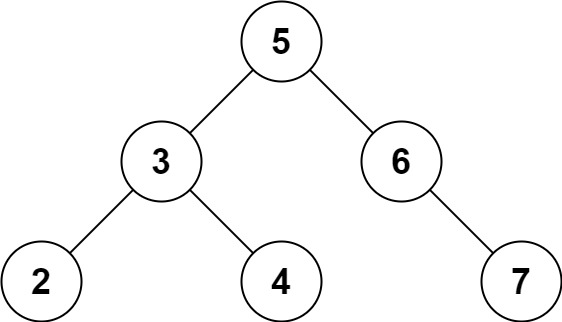``` Input: root = [5,3,6,2,4,null,7], k = 28 Output: false ```

2. Find Target – Solution

The following are two solutions which demonstrates how to get two numbers in a binary search tree equal to target value.

Both solutions use a set to keep track of the items already seen.

Each time a new node is encountered, we subtract the target value from the current node value. If the difference amount from subtracting the two numbers exists in the set, a 2 sum combination exists in the tree

1. Recursive

The following solution uses Depth First Search when looking for the target value.

``` 2. Find Target - Solution - Recursive C# // ============================================================================ // Author: Kenneth Perkins // Date: Oct 9, 2022 // Taken From: http://programmingnotes.org/ // File: Solution.cs // Description: Demonstrates how to get two numbers equal to target value // ============================================================================ public class Solution { public bool FindTarget(TreeNode root, int k) { return Traverse(root, k, new HashSet<int>()); } private bool Traverse(TreeNode node, int k, HashSet<int> seen) { if (node == null) { return false; } // Get remaining value var remaining = k - node.val; // Check if remaining value has been seen if (seen.Contains(remaining)) { return true; } // Add current node value to items seen seen.Add(node.val); // Keep traversing left and right looking for 2 sum return Traverse(node.left, k, seen) || Traverse(node.right, k, seen); } }// http://programmingnotes.org/ 123456789101112131415161718192021222324252627282930 // ============================================================================//    Author: Kenneth Perkins//    Date:   Oct 9, 2022//    Taken From: http://programmingnotes.org///    File:  Solution.cs//    Description: Demonstrates how to get two numbers equal to target value// ============================================================================public class Solution {    public bool FindTarget(TreeNode root, int k) {        return Traverse(root, k, new HashSet<int>());    }     private bool Traverse(TreeNode node, int k, HashSet<int> seen) {        if (node == null) {            return false;        }        // Get remaining value        var remaining = k - node.val;         // Check if remaining value has been seen        if (seen.Contains(remaining)) {            return true;        }        // Add current node value to items seen        seen.Add(node.val);         // Keep traversing left and right looking for 2 sum        return Traverse(node.left, k, seen) || Traverse(node.right, k, seen);    }}// http://programmingnotes.org/ ```

2. Iterative

The following solution uses Breadth First Search when looking for the target value.

``` 2. Find Target - Solution - Iterative C# // ============================================================================ // Author: Kenneth Perkins // Date: Oct 9, 2022 // Taken From: http://programmingnotes.org/ // File: Solution.cs // Description: Demonstrates how to get two numbers equal to target value // ============================================================================ public class Solution { public bool FindTarget(TreeNode root, int k) { if (root == null) { return false; } // Declare stack var stack = new Stack<TreeNode>(); // Keep track of values seen var seen = new HashSet<int>(); // Add root to stack stack.Push(root); // Loop through items on the stack while (stack.Count > 0) { // Get current node var node = stack.Pop(); // Get remaining value var remaining = k - node.val; // Check if remaining value has been seen if (seen.Contains(remaining)) { return true; } else { // Keep traversing left and right looking for 2 sum if (node.left != null) { stack.Push(node.left); } if (node.right != null) { stack.Push(node.right); } } // Add current node value to items seen seen.Add(node.val); } return false; } }// http://programmingnotes.org/ 1234567891011121314151617181920212223242526272829303132333435363738394041424344454647 // ============================================================================//    Author: Kenneth Perkins//    Date:   Oct 9, 2022//    Taken From: http://programmingnotes.org///    File:  Solution.cs//    Description: Demonstrates how to get two numbers equal to target value// ============================================================================public class Solution {    public bool FindTarget(TreeNode root, int k) {        if (root == null) {            return false;        }        // Declare stack        var stack = new Stack<TreeNode>();         // Keep track of values seen        var seen = new HashSet<int>();         // Add root to stack        stack.Push(root);         // Loop through items on the stack        while (stack.Count > 0) {            // Get current node            var node = stack.Pop();             // Get remaining value            var remaining = k - node.val;             // Check if remaining value has been seen            if (seen.Contains(remaining)) {                return true;            } else {                // Keep traversing left and right looking for 2 sum                if (node.left != null) {                    stack.Push(node.left);                }                if (node.right != null) {                    stack.Push(node.right);                }            }            // Add current node value to items seen            seen.Add(node.val);        }        return false;    }}// http://programmingnotes.org/ ```

QUICK NOTES:
The highlighted lines are sections of interest to look out for.

The code is heavily commented, so no further insight is necessary. If you have any questions, feel free to leave a comment below.

Once compiled, you should get this as your output for the example cases:

``` true false ```

## C# || Max Area of Island – How To Find The Maximum Area Of An Island In A Grid Using C#The following is a module with functions which demonstrates how to find the maximum area of an island in a grid using C#.

1. Max Area Of Island – Problem Statement

You are given an m x n binary matrix grid. An island is a group of 1‘s (representing land) connected 4-directionally (horizontal or vertical.) You may assume all four edges of the grid are surrounded by water.

The area of an island is the number of cells with a value 1 in the island.

Return the maximum area of an island in grid. If there is no island, return 0.

Example 1:``` Input: grid = [[0,0,1,0,0,0,0,1,0,0,0,0,0],[0,0,0,0,0,0,0,1,1,1,0,0,0],[0,1,1,0,1,0,0,0,0,0,0,0,0],[0,1,0,0,1,1,0,0,1,0,1,0,0],[0,1,0,0,1,1,0,0,1,1,1,0,0],[0,0,0,0,0,0,0,0,0,0,1,0,0],[0,0,0,0,0,0,0,1,1,1,0,0,0],[0,0,0,0,0,0,0,1,1,0,0,0,0]] Output: 6 Explanation: The answer is not 11, because the island must be connected 4-directionally. ```

Example 2:

``` Input: grid = [[0,0,0,0,0,0,0,0]] Output: 0 ```

2. Max Area Of Island – Solution

The following is a solution which demonstrates how to find the maximum area of an island in a grid.

We want to know the area of each connected shape in the grid, then take the maximum of these.

If we are on a land square and explore every square connected to it 4-directionally (and recursively squares connected to those squares, and so on), then the total number of squares explored will be the area of that connected shape.

To ensure we don’t count squares in a shape more than once, we use ‘seen’ to keep track of squares we haven’t visited before. It will also prevent us from counting the same shape more than once.

``` 2. Max Area Of Island - Solution C# // ============================================================================ // Author: Kenneth Perkins // Date: Jul 28, 2022 // Taken From: http://programmingnotes.org/ // File: Solution.cs // Description: Demonstrates how to find the maximum area in a grid // ============================================================================ public class Solution { public int MaxAreaOfIsland(int[][] grid) { var seen = new bool[grid.Length][]; for (int row = 0; row < seen.Length; ++row) { seen[row] = new bool[grid[row].Length]; } var result = 0; for (int row = 0; row < grid.Length; ++row) { for (int col = 0; col < grid[row].Length; ++col) { result = Math.Max(result, Area(grid, row, col, seen)); } } return result; } private int Area(int[][] grid, int row, int col, bool[][] seen) { if (!IsValidCell(grid, row, col)) { return 0; } if (seen[row][col] || grid[row][col] == 0) { return 0; } seen[row][col] = true; return 1 + // Search Bottom Area(grid, row + 1, col, seen) + // Search Top Area(grid, row - 1, col, seen) + // Search Left Area(grid, row, col - 1, seen) + // Search Right Area(grid, row, col + 1, seen); } private bool IsValidCell(int[][] grid, int row, int col) { return row >= 0 && row < grid.Length && col >= 0 && col < grid[row].Length; } }// http://programmingnotes.org/ 123456789101112131415161718192021222324252627282930313233343536373839404142434445 // ============================================================================//    Author: Kenneth Perkins//    Date:   Jul 28, 2022//    Taken From: http://programmingnotes.org///    File:  Solution.cs//    Description: Demonstrates how to find the maximum area in a grid// ============================================================================public class Solution {    public int MaxAreaOfIsland(int[][] grid) {        var seen = new bool[grid.Length][];        for (int row = 0; row < seen.Length; ++row) {            seen[row] = new bool[grid[row].Length];        }        var result = 0;        for (int row = 0; row < grid.Length; ++row) {            for (int col = 0; col < grid[row].Length; ++col) {                result = Math.Max(result, Area(grid, row, col, seen));            }        }        return result;    }     private int Area(int[][] grid, int row, int col, bool[][] seen) {        if (!IsValidCell(grid, row, col)) {            return 0;        }        if (seen[row][col] || grid[row][col] == 0) {            return 0;        }        seen[row][col] = true;        return 1 +            // Search Bottom            Area(grid, row + 1, col, seen) +            // Search Top            Area(grid, row - 1, col, seen) +            // Search Left            Area(grid, row, col - 1, seen) +            // Search Right            Area(grid, row, col + 1, seen);    }     private bool IsValidCell(int[][] grid, int row, int col) {        return row >= 0 && row < grid.Length && col >= 0 && col < grid[row].Length;    }}// http://programmingnotes.org/ ```

QUICK NOTES:
The highlighted lines are sections of interest to look out for.

The code is heavily commented, so no further insight is necessary. If you have any questions, feel free to leave a comment below.

Once compiled, you should get this as your output for the example cases:

``` 6 0 ```

## C# || Binary Tree Right Side View – How To Get Nodes Ordered Top To Bottom C#The following is a module with functions which demonstrates how to get nodes in a binary tree ordered from top to bottom using C#.

1. Right Side View – Problem Statement

Given the root of a binary tree, imagine yourself standing on the right side of it, return the values of the nodes you can see ordered from top to bottom.

Example 1: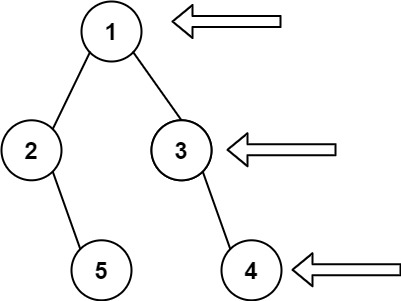``` Input: root = [1,2,3,null,5,null,4] Output: [1,3,4] ```

Example 2:

``` Input: root = [1,null,3] Output: [1,3] ```

Example 3:

``` Input: root = [] Output: [] ```

2. Right Side View – Solution

The following is a solution which demonstrates how to get right side nodes ordered from top to bottom.

This solution uses Depth First Search level order traversal to explore items at each level, and then adds the last node on every layer.

``` 2. Right Side View - Solution C# // ============================================================================ // Author: Kenneth Perkins // Date: Jul 10, 2022 // Taken From: http://programmingnotes.org/ // File: Solution.cs // Description: Demonstrates how to get nodes ordered from top to bottom // ============================================================================ /** * Definition for a binary tree node. * public class TreeNode { * public int val; * public TreeNode left; * public TreeNode right; * public TreeNode(int val=0, TreeNode left=null, TreeNode right=null) { * this.val = val; * this.left = left; * this.right = right; * } * } */ public class Solution { public IList<int> RightSideView(TreeNode root) { var result = new List<int>(); Traverse(root, 0, result); return result; } public void Traverse(TreeNode node, int currentDepth, List<int> result) { if (node == null) { return; } if (currentDepth == result.Count) { result.Add(node.val); } Traverse(node.right, currentDepth + 1, result); Traverse(node.left, currentDepth + 1, result); } }// http://programmingnotes.org/ 1234567891011121314151617181920212223242526272829303132333435363738 // ============================================================================//    Author: Kenneth Perkins//    Date:   Jul 10, 2022//    Taken From: http://programmingnotes.org///    File:  Solution.cs//    Description: Demonstrates how to get nodes ordered from top to bottom// ============================================================================/** * Definition for a binary tree node. * public class TreeNode { *     public int val; *     public TreeNode left; *     public TreeNode right; *     public TreeNode(int val=0, TreeNode left=null, TreeNode right=null) { *         this.val = val; *         this.left = left; *         this.right = right; *     } * } */public class Solution {    public IList<int> RightSideView(TreeNode root) {        var result = new List<int>();        Traverse(root, 0, result);        return result;    }     public void Traverse(TreeNode node, int currentDepth, List<int> result) {        if (node == null) {            return;        }        if (currentDepth == result.Count) {            result.Add(node.val);        }        Traverse(node.right, currentDepth + 1, result);        Traverse(node.left, currentDepth + 1, result);    }}// http://programmingnotes.org/ ```

QUICK NOTES:
The highlighted lines are sections of interest to look out for.

The code is heavily commented, so no further insight is necessary. If you have any questions, feel free to leave a comment below.

Once compiled, you should get this as your output for the example cases:

``` [1,3,4] [1,3] [] ```

## C# || How To Get Total Sum Root To Leaf Binary Numbers In Binary Tree Using C#The following is a module with functions which demonstrates how to get the total sum root to leaf binary numbers in a binary tree using C#.

1. Sum Root To Leaf – Problem Statement

You are given the root of a binary tree where each node has a value 0 or 1. Each root-to-leaf path represents a binary number starting with the most significant bit.

• For example, if the path is 0 -> 1 -> 1 -> 0 -> 1, then this could represent 01101 in binary, which is 13.

For all leaves in the tree, consider the numbers represented by the path from the root to that leaf. Return the sum of these numbers.

The test cases are generated so that the answer fits in a 32-bits integer.

A leaf node is a node with no children.

Example 1: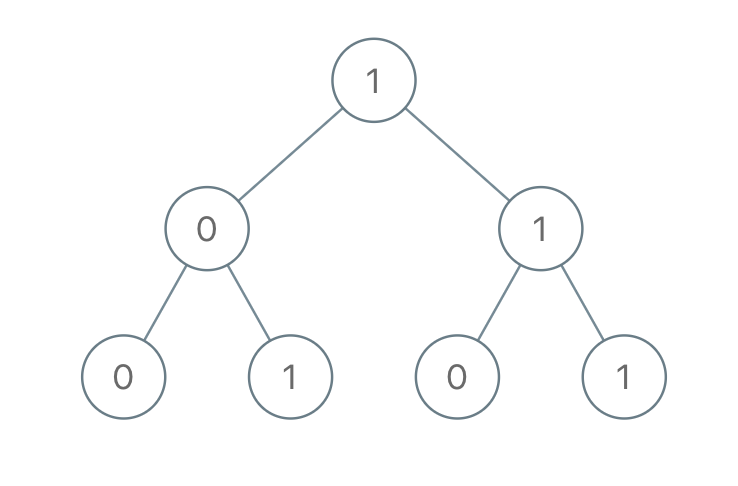``` Input: root = [1,0,1,0,1,0,1] Output: 22 Explanation: (100) + (101) + (110) + (111) = 4 + 5 + 6 + 7 = 22 ```

Example 2:

``` Input: root =  Output: 0 ```

2. Sum Root To Leaf – Solution

The following is a solution which demonstrates how to get the total sum root to leaf binary numbers in a binary tree.

This solution uses Depth First Search to explore items at each level.

``` 2. Sum Root To Leaf - Solution C# // ============================================================================ // Author: Kenneth Perkins // Date: Jan 10, 2022 // Taken From: http://programmingnotes.org/ // File: Solution.cs // Description: Demonstrates how to get sum root to leaf binary numbers // ============================================================================ /** * Definition for a binary tree node. * public class TreeNode { * public int val; * public TreeNode left; * public TreeNode right; * public TreeNode(int val=0, TreeNode left=null, TreeNode right=null) { * this.val = val; * this.left = left; * this.right = right; * } * } */ public class Solution { public int SumRootToLeaf(TreeNode root) { return Traverse(root, 0); } private int Traverse(TreeNode node, int currentSum) { if (node == null) { return 0; } // Calculate current sum currentSum = (currentSum * 2) + node.val; // We have reached a leaf node if (node.left == null && node.right == null) { return currentSum; } // Keep traversing left and right calculating the sum return Traverse(node.left, currentSum) + Traverse(node.right, currentSum); } }// http://programmingnotes.org/ 123456789101112131415161718192021222324252627282930313233343536373839 // ============================================================================//    Author: Kenneth Perkins//    Date:   Jan 10, 2022//    Taken From: http://programmingnotes.org///    File:  Solution.cs//    Description: Demonstrates how to get sum root to leaf binary numbers// ============================================================================/** * Definition for a binary tree node. * public class TreeNode { *     public int val; *     public TreeNode left; *     public TreeNode right; *     public TreeNode(int val=0, TreeNode left=null, TreeNode right=null) { *         this.val = val; *         this.left = left; *         this.right = right; *     } * } */public class Solution {    public int SumRootToLeaf(TreeNode root) {        return Traverse(root, 0);    }    private int Traverse(TreeNode node, int currentSum) {        if (node == null) {            return 0;        }        // Calculate current sum        currentSum = (currentSum * 2) + node.val;         // We have reached a leaf node        if (node.left == null && node.right == null) {            return currentSum;        }        // Keep traversing left and right calculating the sum        return Traverse(node.left, currentSum) + Traverse(node.right, currentSum);    }}// http://programmingnotes.org/ ```

QUICK NOTES:
The highlighted lines are sections of interest to look out for.

The code is heavily commented, so no further insight is necessary. If you have any questions, feel free to leave a comment below.

Once compiled, you should get this as your output for the example cases:

``` 22 0 ```

## C# || Jump Game III – How To Check If You Can Reach Target Value In Array Using C#The following is a module with functions which demonstrates how to check if you can reach a target value in an array using C#.

1. Can Reach – Problem Statement

Given an array of non-negative integers arr, you are initially positioned at start index of the array. When you are at index i, you can jump to i + arr[i] or i – arr[i], check if you can reach to any index with value 0.

Notice that you can not jump outside of the array at any time.

Example 1:

``` Input: arr = [4,2,3,0,3,1,2], start = 5 Output: true Explanation: All possible ways to reach at index 3 with value 0 are: index 5 -> index 4 -> index 1 -> index 3 index 5 -> index 6 -> index 4 -> index 1 -> index 3 ```

Example 2:

``` Input: arr = [4,2,3,0,3,1,2], start = 0 Output: true Explanation: One possible way to reach at index 3 with value 0 is: index 0 -> index 4 -> index 1 -> index 3 ```

Example 3:

``` Input: arr = [3,0,2,1,2], start = 2 Output: false Explanation: There is no way to reach at index 1 with value 0. ```

2. Can Reach – Solution

The following are two solutions which demonstrates how to check if you can reach a target value in an array.

The following solution uses Depth First Search when looking for the target value.

``` 2. Can Reach - DFS - Solution C# // ============================================================================ // Author: Kenneth Perkins // Date: Dec 8, 2021 // Taken From: http://programmingnotes.org/ // File: Solution.cs // Description: Determines if a target value can be reached in an array // ============================================================================ public class Solution { public bool CanReach(int[] arr, int start) { var visited = new bool[arr.Length]; return DFS(arr, start, visited); } public bool DFS(int[] arr, int currentIndex, bool[] visited) { // Make sure parameters are in bounds if (currentIndex < 0 || currentIndex >= arr.Length) { return false; } // Check to see if the index has been visited if (visited[currentIndex]) { return false; } // Check to see if we found the target value if (arr[currentIndex] == 0) { return true; } // Mark current index as visited visited[currentIndex] = true; // Keep searching forwards and backwards var forwards = currentIndex + arr[currentIndex]; var backwards = currentIndex - arr[currentIndex]; return DFS(arr, backwards, visited) || DFS(arr, forwards, visited); } }// http://programmingnotes.org/ 1234567891011121314151617181920212223242526272829303132333435 // ============================================================================//    Author: Kenneth Perkins//    Date:   Dec 8, 2021//    Taken From: http://programmingnotes.org///    File:  Solution.cs//    Description: Determines if a target value can be reached in an array// ============================================================================public class Solution {    public bool CanReach(int[] arr, int start) {        var visited = new bool[arr.Length];        return DFS(arr, start, visited);    }    public bool DFS(int[] arr, int currentIndex, bool[] visited) {        // Make sure parameters are in bounds        if (currentIndex < 0 || currentIndex >= arr.Length) {            return false;        }        // Check to see if the index has been visited        if (visited[currentIndex]) {            return false;        }        // Check to see if we found the target value        if (arr[currentIndex] == 0) {            return true;        }        // Mark current index as visited        visited[currentIndex] = true;         // Keep searching forwards and backwards        var forwards = currentIndex + arr[currentIndex];        var backwards = currentIndex - arr[currentIndex];         return DFS(arr, backwards, visited) || DFS(arr, forwards, visited);    }}// http://programmingnotes.org/ ```

The following solution uses Breadth First Search when looking for the target value.

``` 2. Can Reach - BFS - Solution C# // ============================================================================ // Author: Kenneth Perkins // Date: Dec 8, 2021 // Taken From: http://programmingnotes.org/ // File: Solution.cs // Description: Determines if a target value can be reached in an array // ============================================================================ public class Solution { public bool CanReach(int[] arr, int start) { var visited = new bool[arr.Length]; var queue = new Queue<int>(); queue.Enqueue(start); while (queue.Count > 0) { var currentIndex = queue.Dequeue(); // Check to see if we found the target value if (arr[currentIndex] == 0) { return true; } // Mark current index as visited visited[currentIndex] = true; // Keep searching forwards and backwards var forwards = currentIndex + arr[currentIndex]; var backwards = currentIndex - arr[currentIndex]; var directions = new List<int>{forwards, backwards}; foreach (var direction in directions) { if (direction >= 0 && direction < arr.Length && !visited[direction]) { queue.Enqueue(direction); } } } return false; } }// http://programmingnotes.org/ 1234567891011121314151617181920212223242526272829303132333435363738 // ============================================================================//    Author: Kenneth Perkins//    Date:   Dec 8, 2021//    Taken From: http://programmingnotes.org///    File:  Solution.cs//    Description: Determines if a target value can be reached in an array// ============================================================================public class Solution {    public bool CanReach(int[] arr, int start) {        var visited = new bool[arr.Length];        var queue = new Queue<int>();         queue.Enqueue(start);         while (queue.Count > 0) {            var currentIndex = queue.Dequeue();             // Check to see if we found the target value            if (arr[currentIndex] == 0) {                return true;            }            // Mark current index as visited            visited[currentIndex] = true;             // Keep searching forwards and backwards            var forwards = currentIndex + arr[currentIndex];            var backwards = currentIndex - arr[currentIndex];            var directions = new List<int>{forwards, backwards};             foreach (var direction in directions) {                if (direction >= 0 && direction < arr.Length && !visited[direction]) {                    queue.Enqueue(direction);                }            }        }        return false;    }}// http://programmingnotes.org/ ```

QUICK NOTES:
The highlighted lines are sections of interest to look out for.

The code is heavily commented, so no further insight is necessary. If you have any questions, feel free to leave a comment below.

Once compiled, you should get this as your output for the example cases:

``` true true false ```

## C# || How To Get Total Sum Of Left Leaves In Binary Tree Using C#The following is a module with functions which demonstrates how to get the total sum of left leaves in a binary tree using C#.

1. Sum Of Left Leaves – Problem Statement

Given the root of a binary tree, return the sum of all left leaves.

A leaf node is a node with no children.

Example 1:``` Input: root = [3,9,20,null,null,15,7] Output: 24 Explanation: There are two left leaves in the binary tree, with values 9 and 15 respectively. ```

Example 2:

``` Input: root =  Output: 0 ```

2. Sum Of Left Leaves – Solution

The following are two solutions which demonstrates how to get the total sum of left leaves in a binary tree.

Both solutions use Depth First Search to explore items at each level.

In both solutions, we traverse the left and right side of the tree, keeping track of which node is the ‘left’ node.

When a leaf is encountered and its a node on the left side, we increment the final result with the value of the node on the left side.

Recursive

``` 2. Sum Of Left Leaves - Recursive - Solution C# // ============================================================================ // Author: Kenneth Perkins // Date: Nov 3, 2021 // Taken From: http://programmingnotes.org/ // File: Solution.cs // Description: Demonstrates how to get the sum of left leaves // ============================================================================ /** * Definition for a binary tree node. * public class TreeNode { * public int val; * public TreeNode left; * public TreeNode right; * public TreeNode(int val=0, TreeNode left=null, TreeNode right=null) { * this.val = val; * this.left = left; * this.right = right; * } * } */ public class Solution { public int SumOfLeftLeaves(TreeNode root) { return Traverse(root, false); } private int Traverse(TreeNode node, bool isLeft) { if (node == null) { return 0; } // We have reached a leaf node if (node.left == null && node.right == null) { return isLeft ? node.val : 0; } // Keep traversing left and right looking for left leaves return Traverse(node.left, true) + Traverse(node.right, false); } }// http://programmingnotes.org/ 12345678910111213141516171819202122232425262728293031323334353637 // ============================================================================//    Author: Kenneth Perkins//    Date:   Nov 3, 2021//    Taken From: http://programmingnotes.org///    File:  Solution.cs//    Description: Demonstrates how to get the sum of left leaves// ============================================================================/** * Definition for a binary tree node. * public class TreeNode { *     public int val; *     public TreeNode left; *     public TreeNode right; *     public TreeNode(int val=0, TreeNode left=null, TreeNode right=null) { *         this.val = val; *         this.left = left; *         this.right = right; *     } * } */public class Solution {    public int SumOfLeftLeaves(TreeNode root) {        return Traverse(root, false);    }     private int Traverse(TreeNode node, bool isLeft) {        if (node == null) {            return 0;        }        // We have reached a leaf node        if (node.left == null && node.right == null) {            return isLeft ? node.val : 0;        }        // Keep traversing left and right looking for left leaves        return Traverse(node.left, true) + Traverse(node.right, false);    }}// http://programmingnotes.org/ ```

Iterative

``` 2. Sum Of Left Leaves - Iterative - Solution C# // ============================================================================ // Author: Kenneth Perkins // Date: Nov 3, 2021 // Taken From: http://programmingnotes.org/ // File: Solution.cs // Description: Demonstrates how to get the sum of left leaves // ============================================================================ /** * Definition for a binary tree node. * public class TreeNode { * public int val; * public TreeNode left; * public TreeNode right; * public TreeNode(int val=0, TreeNode left=null, TreeNode right=null) { * this.val = val; * this.left = left; * this.right = right; * } * } */ public class Solution { public int SumOfLeftLeaves(TreeNode root) { if (root == null) { return 0; } // Declare stack var stack = new Stack<KeyValuePair<TreeNode, bool>>(); var result = 0; // Add root to stack stack.Push(new KeyValuePair<TreeNode, bool>(root, false)); // Loop through items on the stack while (stack.Count > 0) { // Get current node and value indicating if its a left node var current = stack.Pop(); var node = current.Key; var isLeft = current.Value; // We have reached a leaf node if (node.left == null && node.right == null) { result += isLeft ? node.val : 0; } else { // Keep traversing left and right looking for left leaves if (node.left != null) { stack.Push(new KeyValuePair<TreeNode, bool>(node.left, true)); } if (node.right != null) { stack.Push(new KeyValuePair<TreeNode, bool>(node.right, false)); } } } return result; } }// http://programmingnotes.org/ 1234567891011121314151617181920212223242526272829303132333435363738394041424344454647484950515253545556 // ============================================================================//    Author: Kenneth Perkins//    Date:   Nov 3, 2021//    Taken From: http://programmingnotes.org///    File:  Solution.cs//    Description: Demonstrates how to get the sum of left leaves// ============================================================================/** * Definition for a binary tree node. * public class TreeNode { *     public int val; *     public TreeNode left; *     public TreeNode right; *     public TreeNode(int val=0, TreeNode left=null, TreeNode right=null) { *         this.val = val; *         this.left = left; *         this.right = right; *     } * } */public class Solution {    public int SumOfLeftLeaves(TreeNode root) {        if (root == null) {            return 0;        }        // Declare stack        var stack = new Stack<KeyValuePair<TreeNode, bool>>();        var result = 0;         // Add root to stack        stack.Push(new KeyValuePair<TreeNode, bool>(root, false));         // Loop through items on the stack        while (stack.Count > 0) {            // Get current node and value indicating if its a left node            var current = stack.Pop();            var node = current.Key;            var isLeft = current.Value;             // We have reached a leaf node            if (node.left == null && node.right == null) {                result += isLeft ? node.val : 0;            } else {                // Keep traversing left and right looking for left leaves                if (node.left != null) {                    stack.Push(new KeyValuePair<TreeNode, bool>(node.left, true));                }                if (node.right != null) {                    stack.Push(new KeyValuePair<TreeNode, bool>(node.right, false));                }            }        }         return result;    }}// http://programmingnotes.org/ ```

QUICK NOTES:
The highlighted lines are sections of interest to look out for.

The code is heavily commented, so no further insight is necessary. If you have any questions, feel free to leave a comment below.

Once compiled, you should get this as your output for the example cases:

``` 24 0 ```

## C# || How To Get Total Sum Root To Leaf Numbers In Binary Tree Using C#The following is a module with functions which demonstrates how to get the total sum root to leaf numbers in a binary tree using C#.

1. Sum Numbers – Problem Statement

You are given the root of a binary tree containing digits from 0 to 9 only.

Each root-to-leaf path in the tree represents a number.

• For example, the root-to-leaf path 1 -> 2 -> 3 represents the number 123.

Return the total sum of all root-to-leaf numbers. Test cases are generated so that the answer will fit in a 32-bit integer.

A leaf node is a node with no children.

Example 1: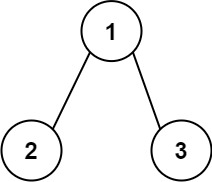``` Input: root = [1,2,3] Output: 25 Explanation: The root-to-leaf path 1->2 represents the number 12. The root-to-leaf path 1->3 represents the number 13. Therefore, sum = 12 + 13 = 25. ```

Example 2:``` Input: root = [4,9,0,5,1] Output: 1026 Explanation: The root-to-leaf path 4->9->5 represents the number 495. The root-to-leaf path 4->9->1 represents the number 491. The root-to-leaf path 4->0 represents the number 40. Therefore, sum = 495 + 491 + 40 = 1026. ```

2. Sum Numbers – Solution

The following are two solutions which demonstrates how to get the total sum root to leaf numbers in a binary tree.

Both solutions use Depth First Search to explore items at each level.

Recursive

``` 2. Sum Numbers - Recursive - Solution C# // ============================================================================ // Author: Kenneth Perkins // Date: Nov 3, 2021 // Taken From: http://programmingnotes.org/ // File: Solution.cs // Description: Demonstrates how to get sum root to leaf numbers // ============================================================================ /** * Definition for a binary tree node. * public class TreeNode { * public int val; * public TreeNode left; * public TreeNode right; * public TreeNode(int val=0, TreeNode left=null, TreeNode right=null) { * this.val = val; * this.left = left; * this.right = right; * } * } */ public class Solution { public int SumNumbers(TreeNode root) { return Traverse(root, 0); } private int Traverse(TreeNode node, int currentSum) { if (node == null) { return 0; } // Calculate current sum currentSum = (currentSum * 10) + node.val; // We have reached a leaf node if (node.left == null && node.right == null) { return currentSum; } // Keep traversing left and right calculating the sum return Traverse(node.left, currentSum) + Traverse(node.right, currentSum); } }// http://programmingnotes.org/ 123456789101112131415161718192021222324252627282930313233343536373839404142 // ============================================================================//    Author: Kenneth Perkins//    Date:   Nov 3, 2021//    Taken From: http://programmingnotes.org///    File:  Solution.cs//    Description: Demonstrates how to get sum root to leaf numbers// ============================================================================/** * Definition for a binary tree node. * public class TreeNode { *     public int val; *     public TreeNode left; *     public TreeNode right; *     public TreeNode(int val=0, TreeNode left=null, TreeNode right=null) { *         this.val = val; *         this.left = left; *         this.right = right; *     } * } */public class Solution {    public int SumNumbers(TreeNode root) {        return Traverse(root, 0);    }     private int Traverse(TreeNode node, int currentSum) {        if (node == null) {            return 0;        }         // Calculate current sum        currentSum = (currentSum * 10) + node.val;         // We have reached a leaf node        if (node.left == null && node.right == null) {            return currentSum;        }         // Keep traversing left and right calculating the sum        return Traverse(node.left, currentSum) + Traverse(node.right, currentSum);    }}// http://programmingnotes.org/ ```

Iterative

``` 2. Sum Numbers - Iterative - Solution C# // ============================================================================ // Author: Kenneth Perkins // Date: Nov 3, 2021 // Taken From: http://programmingnotes.org/ // File: Solution.cs // Description: Demonstrates how to get sum root to leaf numbers // ============================================================================ /** * Definition for a binary tree node. * public class TreeNode { * public int val; * public TreeNode left; * public TreeNode right; * public TreeNode(int val=0, TreeNode left=null, TreeNode right=null) { * this.val = val; * this.left = left; * this.right = right; * } * } */ public class Solution { public int SumNumbers(TreeNode root) { if (root == null) { return 0; } // Declare stack var stack = new Stack<KeyValuePair<TreeNode, int>>(); var result = 0; // Add root to stack stack.Push(new KeyValuePair<TreeNode, int>(root, 0)); // Loop through items on the stack while (stack.Count > 0) { // Get current node and running sum var current = stack.Pop(); var node = current.Key; var currentSum = current.Value; // Calculate current sum currentSum = (currentSum * 10) + node.val; // We have reached a leaf node if (node.left == null && node.right == null) { result += currentSum; } else { // Keep traversing left and right calculating the sum if (node.left != null) { stack.Push(new KeyValuePair<TreeNode, int>(node.left, currentSum)); } if (node.right != null) { stack.Push(new KeyValuePair<TreeNode, int>(node.right, currentSum)); } } } return result; } }// http://programmingnotes.org/ 12345678910111213141516171819202122232425262728293031323334353637383940414243444546474849505152535455565758 // ============================================================================//    Author: Kenneth Perkins//    Date:   Nov 3, 2021//    Taken From: http://programmingnotes.org///    File:  Solution.cs//    Description: Demonstrates how to get sum root to leaf numbers// ============================================================================/** * Definition for a binary tree node. * public class TreeNode { *     public int val; *     public TreeNode left; *     public TreeNode right; *     public TreeNode(int val=0, TreeNode left=null, TreeNode right=null) { *         this.val = val; *         this.left = left; *         this.right = right; *     } * } */public class Solution {    public int SumNumbers(TreeNode root) {        if (root == null) {            return 0;        }        // Declare stack        var stack = new Stack<KeyValuePair<TreeNode, int>>();        var result = 0;         // Add root to stack        stack.Push(new KeyValuePair<TreeNode, int>(root, 0));         // Loop through items on the stack        while (stack.Count > 0) {            // Get current node and running sum            var current = stack.Pop();            var node = current.Key;            var currentSum = current.Value;             // Calculate current sum            currentSum = (currentSum * 10) + node.val;             // We have reached a leaf node            if (node.left == null && node.right == null) {                result += currentSum;            } else {                // Keep traversing left and right calculating the sum                if (node.left != null) {                    stack.Push(new KeyValuePair<TreeNode, int>(node.left, currentSum));                }                if (node.right != null) {                    stack.Push(new KeyValuePair<TreeNode, int>(node.right, currentSum));                }            }        }        return result;    }}// http://programmingnotes.org/ ```

QUICK NOTES:
The highlighted lines are sections of interest to look out for.

The code is heavily commented, so no further insight is necessary. If you have any questions, feel free to leave a comment below.

Once compiled, you should get this as your output for the example cases:

``` 25 1026 ```

## C# || Unique Paths III – How To Get The Number Of Paths From Start To End In Grid Matrix Using C#The following is a module with functions which demonstrates how to get the number of paths from start to end in a grid matrix using C#.

1. Unique Paths III – Problem Statement

You are given an m x n integer array grid where grid[i][j] could be:

• 1 representing the starting square. There is exactly one starting square.
• 2 representing the ending square. There is exactly one ending square.
• 0 representing empty squares we can walk over.
• -1 representing obstacles that we cannot walk over.

Return the number of 4-directional walks from the starting square to the ending square, that walk over every non-obstacle square exactly once.

Example 1: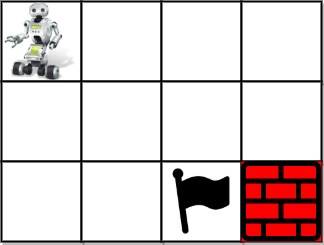``` Input: grid = [[1,0,0,0],[0,0,0,0],[0,0,2,-1]] Output: 2 Explanation: We have the following two paths: 1. (0,0),(0,1),(0,2),(0,3),(1,3),(1,2),(1,1),(1,0),(2,0),(2,1),(2,2) 2. (0,0),(1,0),(2,0),(2,1),(1,1),(0,1),(0,2),(0,3),(1,3),(1,2),(2,2) ```

Example 2: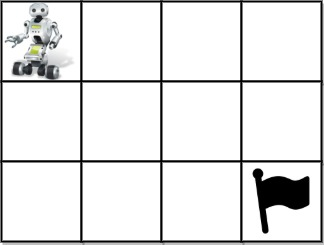``` Input: grid = [[1,0,0,0],[0,0,0,0],[0,0,0,2]] Output: 4 Explanation: We have the following four paths: 1. (0,0),(0,1),(0,2),(0,3),(1,3),(1,2),(1,1),(1,0),(2,0),(2,1),(2,2),(2,3) 2. (0,0),(0,1),(1,1),(1,0),(2,0),(2,1),(2,2),(1,2),(0,2),(0,3),(1,3),(2,3) 3. (0,0),(1,0),(2,0),(2,1),(2,2),(1,2),(1,1),(0,1),(0,2),(0,3),(1,3),(2,3) 4. (0,0),(1,0),(2,0),(2,1),(1,1),(0,1),(0,2),(0,3),(1,3),(1,2),(2,2),(2,3) ```

Example 3:``` Input: grid = [[0,1],[2,0]] Output: 0 Explanation: There is no path that walks over every empty square exactly once. Note that the starting and ending square can be anywhere in the grid. ```

2. Unique Paths III – Solution

The following is a solution which demonstrates how to get the number of paths from start to end in a grid matrix.

This solution uses Depth First Search when looking for paths.

In this solution, we find the starting coordinates and count the number of empty cells.

Then we do DFS, marking the visited positions and keeping track of the number of steps. If we reach the end and the number of steps matches the number of empty cells, we found a path.

``` 2. Unique Paths III - Solution C# // ============================================================================ // Author: Kenneth Perkins // Date: Nov 2, 2021 // Taken From: http://programmingnotes.org/ // File: Solution.cs // Description: Demonstrates how to get total paths in a grid matrix // ============================================================================ public class Solution { private int START = 1; private int END = 2; private int EMPTY = 0; private int OBSTACLE = -1; public int UniquePathsIII(int[][] grid) { // Include the starting position in the empty cell count var emptyCellCount = 1; // The row/col coordinates of the starting point var startRow = -1; var startCol = -1; for (int row = 0; row < grid.Length; ++row) { for (int col = 0; col < grid[row].Length; ++col) { // Get empty cell count if (grid[row][col] == EMPTY) { ++emptyCellCount; // Get the starting coordinates } else if (grid[row][col] == START) { startRow = row; startCol = col; } } } // Return total paths to the end return DFS(grid, startRow, startCol, emptyCellCount); } private int DFS(int[][] grid, int row, int col, int emptyCellCount) { // Make sure parameters are in bounds if (row < 0 || row >= grid.Length || col < 0 || col >= grid[row].Length) { return 0; } // Obstacle encountered or path already visited if (grid[row][col] == OBSTACLE) { return 0; } // We have reached the end if (grid[row][col] == END) { // Check to see if all empty cells have been used return emptyCellCount == 0 ? 1 : 0; } // Save the old value var oldValue = grid[row][col]; // Mark path as visited grid[row][col] = OBSTACLE; // Get the path count in right, left, top, bottom direction var pathCount = // Search Right DFS(grid, row, col + 1, emptyCellCount - 1) + // Search Left DFS(grid, row, col - 1, emptyCellCount - 1) + // Search Top DFS(grid, row - 1, col, emptyCellCount - 1) + // Search Bottom DFS(grid, row + 1, col, emptyCellCount - 1); // Unmark path as visited grid[row][col] = oldValue; return pathCount; } }// http://programmingnotes.org/ 1234567891011121314151617181920212223242526272829303132333435363738394041424344454647484950515253545556575859606162636465666768697071727374757677 // ============================================================================//    Author: Kenneth Perkins//    Date:   Nov 2, 2021//    Taken From: http://programmingnotes.org///    File:  Solution.cs//    Description: Demonstrates how to get total paths in a grid matrix// ============================================================================public class Solution {    private int START = 1;    private int END = 2;    private int EMPTY = 0;    private int OBSTACLE = -1;     public int UniquePathsIII(int[][] grid) {        // Include the starting position in the empty cell count        var emptyCellCount = 1;         // The row/col coordinates of the starting point        var startRow = -1;        var startCol = -1;         for (int row = 0; row < grid.Length; ++row) {            for (int col = 0; col < grid[row].Length; ++col) {                // Get empty cell count                if (grid[row][col] == EMPTY) {                    ++emptyCellCount;                 // Get the starting coordinates                } else if (grid[row][col] == START) {                    startRow = row;                    startCol = col;                }            }        }         // Return total paths to the end        return DFS(grid, startRow, startCol, emptyCellCount);    }     private int DFS(int[][] grid, int row, int col, int emptyCellCount) {        // Make sure parameters are in bounds        if (row < 0 || row >= grid.Length || col < 0 || col >= grid[row].Length) {            return 0;        }        // Obstacle encountered or path already visited        if (grid[row][col] == OBSTACLE) {            return 0;        }        // We have reached the end        if (grid[row][col] == END) {            // Check to see if all empty cells have been used            return emptyCellCount == 0 ? 1 : 0;        }         // Save the old value        var oldValue = grid[row][col];         // Mark path as visited        grid[row][col] = OBSTACLE;         // Get the path count in right, left, top, bottom direction        var pathCount =            // Search Right            DFS(grid, row, col + 1, emptyCellCount - 1) +            // Search Left            DFS(grid, row, col - 1, emptyCellCount - 1) +            // Search Top            DFS(grid, row - 1, col, emptyCellCount - 1) +            // Search Bottom            DFS(grid, row + 1, col, emptyCellCount - 1);         // Unmark path as visited        grid[row][col] = oldValue;         return pathCount;    }}// http://programmingnotes.org/ ```

QUICK NOTES:
The highlighted lines are sections of interest to look out for.

The code is heavily commented, so no further insight is necessary. If you have any questions, feel free to leave a comment below.

Once compiled, you should get this as your output for the example cases:

``` 2 4 0 ```